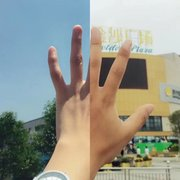{meta: 原调: 1=G} {meta: 选调: 1=G} {meta: Capo=0} {comment: 前奏(笛子)} [G] [Em] [G] [Em] [C] [G] [Em] [C] [G] [C] [D] {comment: 主歌 A} [G] 这个冬天雪还不下 [Em] 站在路上眼睛不眨 [G] 我的心跳还很温柔 [Em] 你该表扬我说[C]今天还很听话 [G] 我的衣服有些大了 [Em] 你说我看起来[C]挺嘎 [G] 我知道我站在[C]人群里 [C] 挺[D]傻 [D] 2/4 [F] 我的爹他总在喝酒是个[C]混球 再[F]死之前他不会再伤心不[C]再动拳头 [F] 他坐在楼梯上也[C]已经苍老 已不是[D]对手 {comment: 主歌 B} [G] 感到要被欺骗之前 [Em] 自己总是做不伟大 [G]听不到他们说什么 [Em] 只是想人要孤[C]单容易尴尬 [G]面对外前面的人群 [Em] 我得穿过而且[C]潇洒 [G] 我知道你在旁[C]边看着 [C] 挺[D]假 [D] 2/4 [F] 姐姐我看见你眼里的[C]泪水 [F] 你想忘掉那侮辱你的男人[C]到底是谁 [F] 他们告诉我女人很温柔[C]很爱流泪 说这[D]很美 {comment: 副歌} {start_of_chorus} 噢[G]姐姐 我想[Em]回家 牵着[C]我的手 我有些[D]困了 噢[G]姐姐 带我[Em]回家 牵着[C]我的手 你不要[D]害怕 {end_of_chorus} {comment: 主歌 A} [F] 我的爹他总在喝酒是个[C]混球 再[F]死之前他不会再伤心不[C]再动拳头 [F] 他坐在楼梯上也[C]已经苍老 已不是[D]对手 {comment: 副歌} {start_of_chorus} 噢[G]姐姐 我想[Em]回家 牵着[C]我的手 我有些[D]困了 噢[G]姐姐 带我[Em]回家 牵着[C]我的手 你不要[D]害怕 噢[G]姐姐 带我[Em]回家 牵着[C]我的手 你不要[D]害怕 噢[G]姐姐 我想[Em]回家 牵着[C]我的手 我有些[D]困了 {end_of_chorus} {comment: 尾奏(笛子)} {comment: 听作者演唱} VX:ZT35075882 荔枝直播：35075882 唱吧：WQ373439852 全民K歌：867618220 {title:姐姐（张楚原版顺序）} {artist:张楚} {author:沙滩裤}